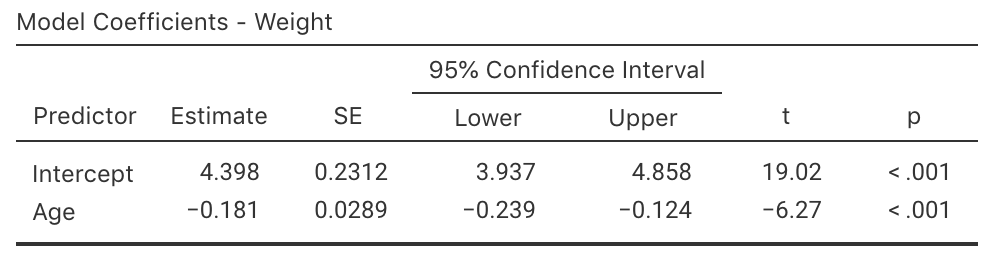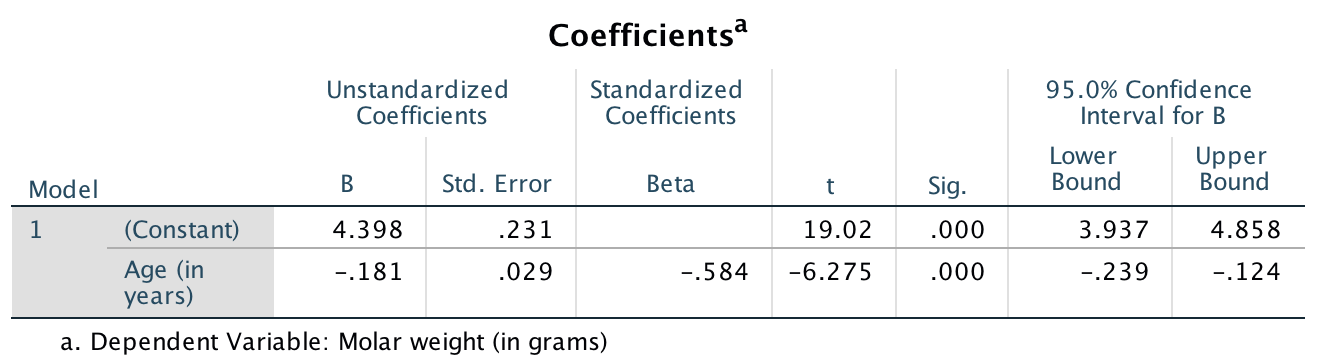## 35.7 Confidence intervals

Reporting the CI for the slope is also useful, which can be obtained from software or computed manually.

Think 35.4 (Approximate 95% CI) Using the output (jamovi: Fig. 35.7; SPSS: Fig. 35.8), what is the approximate 95% CI for $$\beta_1$$?

CIs have the form $\text{statistic} \pm ( \text{multiplier} \times \text{standard error}),$ The multiplier is two for an approximate 95% CI, so (using the standard error reported by the software), we obtain $$-0.181 \pm (2\times 0.029)$$, or $$-0.181 \pm 0.058$$, or from $$-0.239$$ to $$-0.123$$.

Software can be asked to produce exact CIs too (jamovi: Fig. 35.11; SPSS: Fig. 35.12). The approximate and exact 95% CIs are very similar.FIGURE 35.11: jamovi output for the red-deer data, including the CIsFIGURE 35.12: SPSS output for the red-deer data, including the CIs

We write:

The sample presents very strong evidence ($$P < 0.001$$; $$t = -6.275$$) of a relationship between age and the weight of molars in male red deer (slope: $$-0.181$$; $$n= 78$$; 95% CI from $$-0.239$$ to $$-0.124$$) in the population.

Example 35.5 (Emergency department patients) In Example 35.4, the jamovi output does not give the 95% CI for the slope.

However, since CIs have the form $\text{statistic} \pm ( \text{multiplier} \times \text{standard error}),$ the CI is easily computed: $-0.34790 \pm (2\times 0.046672),$
or $$-0.34790 \pm 0.093344$$. This is equivalent to $$-0.441$$ to $$-0.255$$.

Combining with information from Example 35.4, we write:

The sample presents very strong evidence ($$P < 0.001$$; $$t = -7.45$$) of a relationship between the mean number of ED patients and the numbers of days after welfare distribution (slope: $$-0.348$$; $$n = 30$$; 95% CI from $$-0.441$$ to $$-0.255$$) in the population.